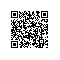# Javascript高级程序设计第二版第七章匿名函数--笔记

function functionName(){};

var functionName = function(){};

## 7.1 递归

arguments.callee是一个指向正在执行的函数的指针，可以实现函数的递归调用

function factorial(num){

if(num <= 1){

return 1;

} else {

return num * arguments.callee(num - 1);

}

}

var anotherFactorial = factorial;

factorial = null;

## 7.2 闭包

function fn(propertyName){

return function(object1){

var v1 = object1[propertyName]; //访问了外部变量propertyName变量

}

}

### 7.2.1 闭包与变量

function createFunctions(){

var result = [];

for(var i=0; i<10; i++){

result[i] = function(num){

return function(){

return num;

}

}(i);

}

}

var funcs = createFunctions();

for(var i=0; i<funcs.length; i++){

document.write(funcs[i] + ‘<br />’); //output 0,1,2,3…9

}

### 7.2.2 this对象

this对象是在运行时基于函数的执行环境绑定的：在全局函数中，this等于window,而当函数被作为某个对象的方法调用时，this等于那个对象。不过，匿名函数的执行环境具有全局性，因此对象通常指向window.

### 7.2.3 内存泄漏

function assignHandler(){

var element = document.getElementById(‘someElement’);

var id = element.id;

element.onclick = function(){

};

element = null; //变量设置为null，才能解除对DOM对象的引用，顺利减少引用数，确保正常回收其占用的内存

}

## 7.3 模仿块级作用域

(function(){

//这里是块级作用域

})();

var someFunction = function(){};

someFunction();

function(){}();

## 7.4 私有变量

function MyObject(){

//私有变量

var privateVariable = 10;

function privateFunction(){

return false;

}

//特权方法

this.publicMethod = function(){

privateVariable ++;

return privateFunction();

}

}

### 7.4.1 静态私有变量

(function(){

var privateVariable = 10;

function privateFunction(){

}

Myobject = function(){

}

//公有/特权方法

Myobject.prototype.publicMethod = function(){

privateVariable ++;

return privateFunction();

}

})()

### 7.4.2 模块模式

var singleton = {

name: value,

method: function(){

//这里是方法

}

}

var singleton = function(){

//私有变量和私有函数

var privateVariable = 10;

function privateFunction(){}

//特权/公有方法和属性

return {

publicProperty: true,

publicMethod: function(){

privateVariable++;

return privateFunction();

}

}

}

### 7.4.3 增强的模块模式

var singleton = function(){

//私有变量和私有函数

var privateVariable = 10;

function privateFunction(){

return false;

}

//创建对象

var object = new CustomType();

//添加特权/仅有属性和方法

object.publicProperty = true;

object.publicMethod = function(){

privateVariable++;

return privateFunction();

};

return object;

}使用钉钉扫一扫加入圈子
+ 订阅